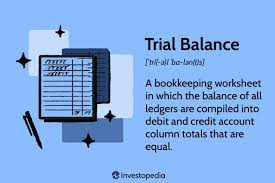Microsoft Excel knowledge is not enough if not practised often. The more you practice, the better you get and the more you learn.

I just learnt some new applications in Excel which made me develop an interest in acquiring more knowledge of the program. I would like to share with you briefly about Excel in this article.

In case you are wondering what Excel is, let me give a background introduction.

Excel is a software program created by Microsoft that uses spreadsheets to organize numbers and data. It organizes data in columns and rows which can be manipulated using formulas to perform mathematical functions on the data.

Excel is mainly used to organize and analyze data. Other uses are listed below:

• Data Entry
• Data Management
• Programming
• Accounting and Financial analysis

Excel is used extensively in finance and accounting functions. For example, budgeting, forecasting, and other accounting functions are mostly done in Excel spreadsheets. This implies that Accountants, analysts, and many other professionals rely mostly on Excel to perform their daily job functions.

Some terminologies used in Excel are:

Rows: These are the horizontal lines in the worksheet which are identified by numbers. Total rows in a worksheet are 1,048,576.

Columns: These are the vertical lines in the worksheet which are identified by letters of the alphabet. The total columns in a worksheet are 16,384.

Cell: This is the intersection where a row and a column meet on a spreadsheet. It is identified by its’s cell address.

Worksheet: This is a page in an Excel workbook. It is a collection of cells organized in rows and columns.

Workbook: This is a spreadsheet document that contains more than one worksheet.

Chart: This is a graphical representation of data.

Data range: This is a list of data

Formula: These are calculations performed on data in a worksheet

There are seven basic rules in Excel that should be taken note of:

1. Avoid empty rows
2. Avoid empty columns
3. Always maintain one row of header
4. There should be no total or subtotal on the datasheet.
5. There should be no obstruction around the total.
6. All dates must be on a single column.
7. All categories/variables must be on a single column.

Excel shortcuts: These are some important time-saving shortcuts used in Excel. They may come very useful at one point or the other while using Excel, hence, it is advisable to learn a handful of them. Examples are listed as follows:

In conclusion, having a good knowledge of Microsoft Excel gives you lots of advantages in data analytics. It reduces time wastage in analyzing to produce a timely report for timely decision making.

Also, as a skilled Excel user, you get lots of job opportunities.

I believe you have learnt a couple of things in Excel already. Watch out for more updates that I will be sharing soon.

#MMBA3## Writing Accounting exams😁

in## Handling Crisis 😒

in